# Lyusternik-Shnirel'man-Borsuk covering theorem

(diff) ← Older revision | Latest revision (diff) | Newer revision → (diff)

A theorem usually stated as follows:

1) Each closed coveringof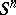contains at least one set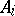with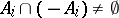.

Contrary to the equivalent Borsuk–Ulam theorem, it seems to be not common to use the same name also for the following equivalent symmetric versions:

2) Letbe closed sets with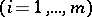. If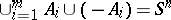, then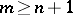.

3)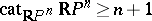[a2].

In all these results, the estimates are optimal (in 3), in fact, equality holds). It is worth mentioning that 2) gave the motivation for the notion of the genus of a set symmetric with respect to a free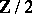-action.

For other equivalent versions and for generalizations to coverings involving other symmetries (e.g. with respect to free-actions), cf. [a3] and the references therein.

One major field of applications are estimates of the number of critical points of even functionals; this can be used, e.g., in the theory of differential equations.

How to Cite This Entry:
Lyusternik-Shnirel'man-Borsuk covering theorem. Encyclopedia of Mathematics. URL: http://encyclopediaofmath.org/index.php?title=Lyusternik-Shnirel%27man-Borsuk_covering_theorem&oldid=18675
This article was adapted from an original article by H. Steinlein (originator), which appeared in Encyclopedia of Mathematics - ISBN 1402006098. See original article